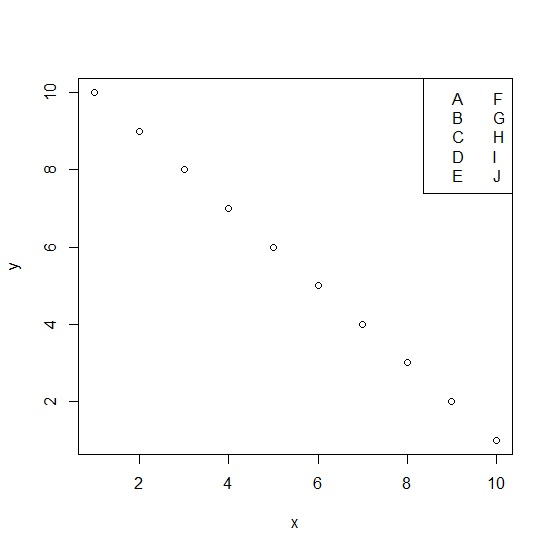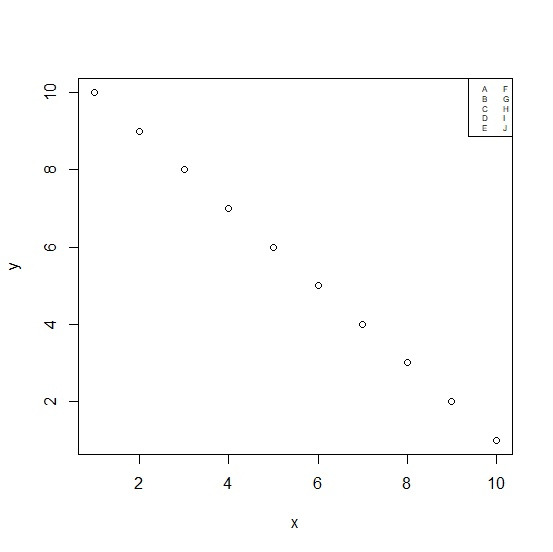# How to reduce the size of the area covered by legend in R for a plot created by using plot function?

By default, the area covered by legends for a plot created by using plot function is of full size that is 1 (the area size has a range of 0 to 1, where 1 refers to the full size and 0 refers to none). To reduce the size, we can use cex argument with the legend function as shown in the below example.

## Example

Consider the below vectors and the plot created between these two vectors −

x<-1:10
y<-10:1 plot((x,y) legend("topright",legend=LETTERS[1:10],ncol=2)

## OutputNow to create the plot with reduced size of the legend values and the legend area, we need to use the following codes −

x<-1:10
y<-10:1
plot(x,y) legend("topright",legend=LETTERS[1:10],ncol=2,cex=0.50)

## Output